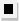Chapter 13.CR, Problem 69E### Algebra and Trigonometry (MindTap ...

4th Edition
James Stewart + 2 others
ISBN: 9781305071742

#### Solutions

Chapter
Section### Algebra and Trigonometry (MindTap ...

4th Edition
James Stewart + 2 others
ISBN: 9781305071742
Textbook Problem

# 6 7 - 6 9Mathematical Induction Use mathematical induction to prove that the formula is true for all natural numbers n . ( 1 + 1 1 ) ( 1 + 1 2 ) ( 1 + 1 3 ) ⋅ ⋅ ⋅ ( 1 + 1 n ) = n + 1

To determine

To prove:

The expression (1+11)(1+12)(1+13)(1+1n)=n+1 using mathematical induction.

Explanation

Given:

The expression is given as,

(1+11)(1+12)(1+13)(1+1n)=n+1...(1)

Approach:

Prove basic step for n=1. Assume the formula is true for some natural number n1 and show that the formula is true for n+1.

Calculation:

Denote the given expression by P(n).

Step 1:

1+11=1+1

This proves Step 1.

Step 2:

Assume that P(n) is true for some n1. This is called the induction hypothesis.

Show that P(n+1) is true

### Still sussing out bartleby?

Check out a sample textbook solution.

See a sample solution

#### The Solution to Your Study Problems

Bartleby provides explanations to thousands of textbook problems written by our experts, many with advanced degrees!

Get Started